83

20

17

12

# GraphRecipes

The repository formerly known as PlotRecipes### Primary author: Thomas Breloff (@tbreloff)

This repo maintains a collection of recipes for graph analysis, and is a reduced and refactored version of the previous PlotRecipes. It uses the powerful machinery of Plots and RecipesBase to turn simple transformations into flexible visualizations.

# Examples

``````using GraphRecipes
using Plots

const n = 15
const A = Float64[ rand() < 0.5 ? 0 : rand() for i=1:n, j=1:n]
for i=1:n
A[i, 1:i-1] = A[1:i-1, i]
end

graphplot(A,
node_weights = 1:n,
nodecolor = range(colorant"yellow", stop=colorant"red", length=n),
names = 1:n,
linecolor = :darkgrey,
)
``````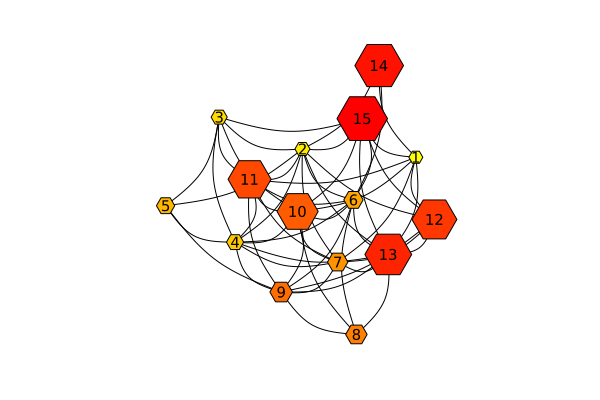Now plot the graph in three dimensions.

``````graphplot(A,
node_weights = 1:n,
markercolor = :darkgray,
dim = 3,
markersize = 5,
linecolor = :darkgrey,
linealpha = 0.5
)
``````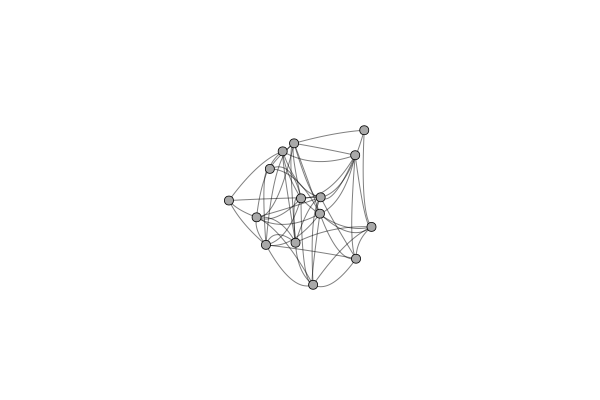#### Arc and chord diagrams

``````using LinearAlgebra
using SparseArrays
using GraphRecipes
using Plots

plot(
method=:chorddiagram,
names=[text(string(i), 8) for i in 1:8],
linecolor=:black,
fillcolor=:lightgray),

method=:arcdiagram,
markersize=3,
linecolor=:black,
markercolor=:black)
)
``````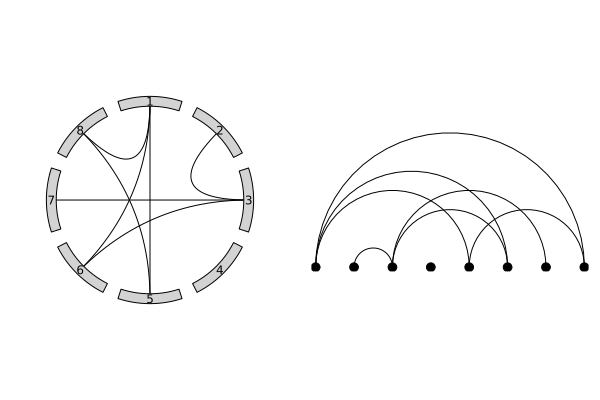#### Julia code -- AST

``````using GraphRecipes
using Plots
default(size=(1000, 1000))

code = :(
function mysum(list)
out = 0
for value in list
out += value
end
out
end
)

plot(code, fontsize=12, shorten=0.01, axis_buffer=0.15, nodeshape=:rect)
``````#### Julia Type Trees

``````using GraphRecipes
using Plots
default(size=(1000, 1000))

plot(AbstractFloat, method=:tree, fontsize=10, nodeshape=:ellipse)
``````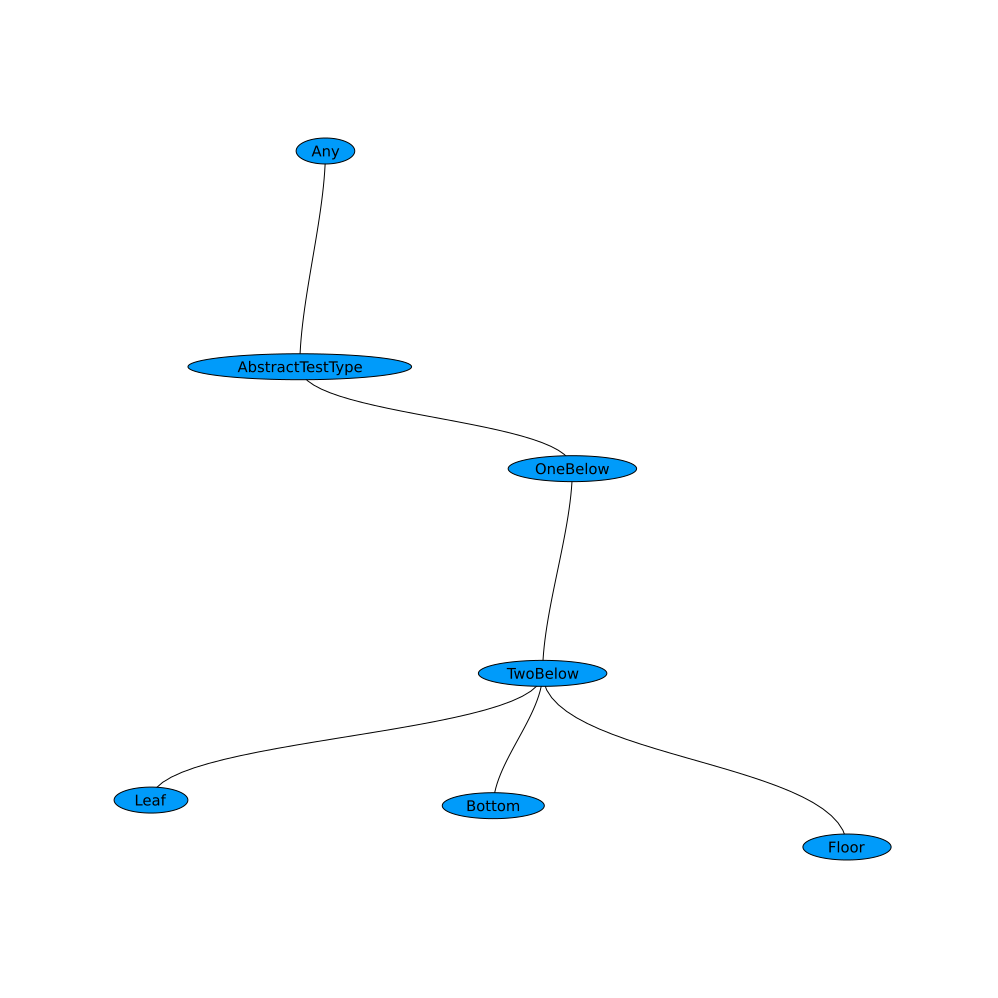#### `AbstractTrees` Trees

``````using AbstractTrees

AbstractTrees.children(d::Dict) = [p for p in d]
AbstractTrees.children(p::Pair) = AbstractTrees.children(p)
function AbstractTrees.printnode(io::IO, p::Pair)
str = isempty(AbstractTrees.children(p)) ? string(p, ": ", p) : string(p, ": ")
print(io, str)
end

d = Dict(:a => 2,:d => Dict(:b => 4,:c => "Hello"),:e => 5.0)

using GraphRecipes
using Plots
default(size=(1000, 1000))

plot(TreePlot(d), method=:tree, fontsize=10, nodeshape=:ellipse)
``````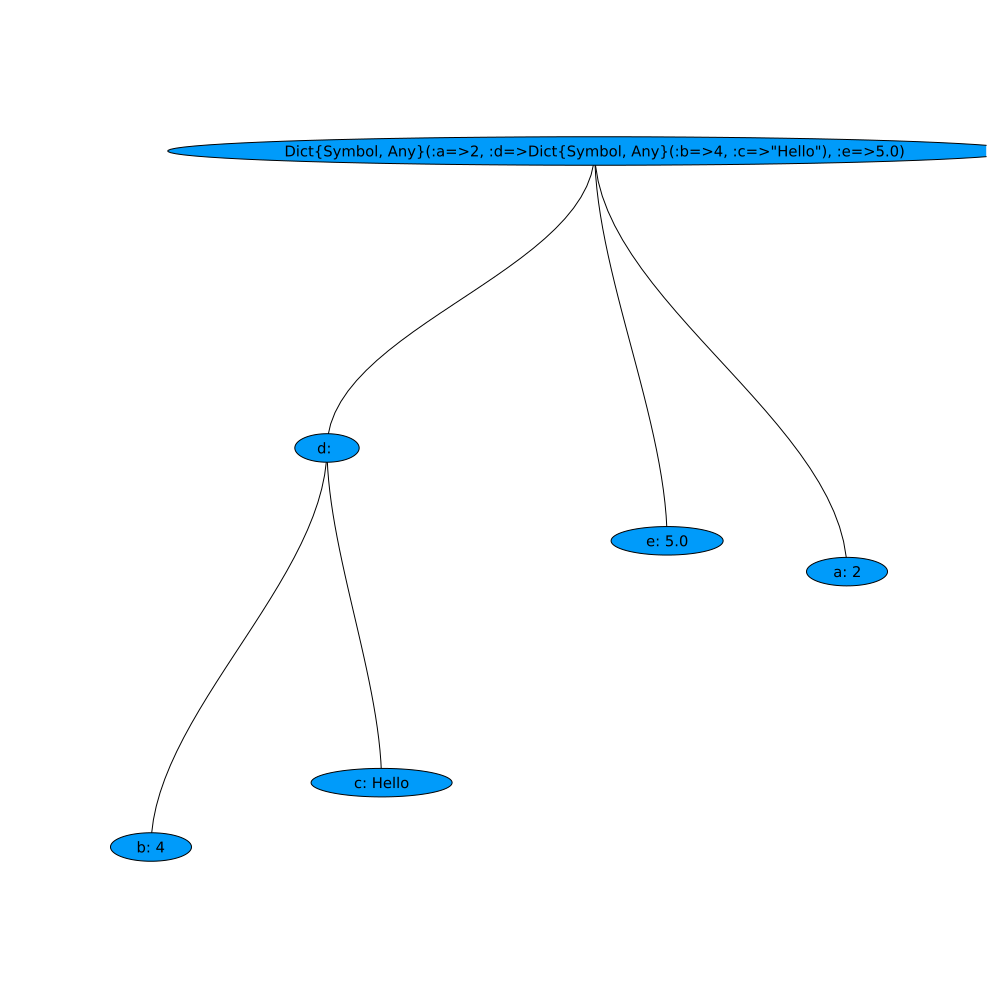05/07/2016

22 days ago

157 commits